# Frequency Polygon Worksheet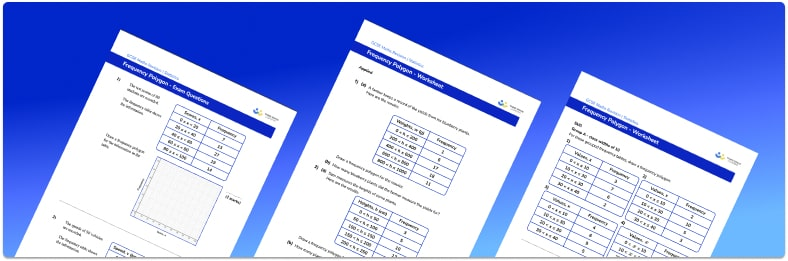• Section 1 of the frequency polygon worksheet contains 12 skills-based frequency polygon questions, in 3 groups to support differentiation
• Section 2 contains 3 applied frequency polygon questions with a mix of worded problems and deeper problem solving questions
• Section 3 contains 3 foundation and higher level GCSE exam style frequency polygon questions
• Answers and a mark scheme for all frequency polygon questions are provided
• Questions follow variation theory with plenty of opportunities for students to work independently at their own level
• All questions created by fully qualified expert secondary maths teachers
• Suitable for GCSE maths revision for AQA, OCR and Edexcel exam boards

• This field is for validation purposes and should be left unchanged.

You can unsubscribe at any time (each email we send will contain an easy way to unsubscribe). To find out more about how we use your data, see our privacy policy.

### Frequency polygon at a glance

There are a few different frequency diagrams for grouped data studied at GCSE, these include cumulative frequency graphs, histograms and frequency polygons. In order to construct a frequency polygon we start with a set of data in a grouped frequency table. The midpoints of each class interval are calculated and the data is plotted on a graph with the category groups on the x axis and frequency on the y axis. Data is plotted using the midpoints of the class intervals, and points are joined with straight line segments.

Frequency polygons are particularly useful when comparing two sets of data,  for example, the heights of a number of students in different age groups. We can use frequency polygons to identify the modal class interval, it can be found by identifying the highest peak on the frequency polygon as this represents the class that occurs the most.

It is important that students are confident with using inequality notation for class intervals, particularly when working with fractions and decimals.

Looking forward, students can then progress to additional Statistics worksheets, for example a histograms worksheet orFor more teaching and learning support on Statistics our GCSE maths lessons provide step by step support for all GCSE maths concepts.

## Related worksheets

X And Y Axis Worksheet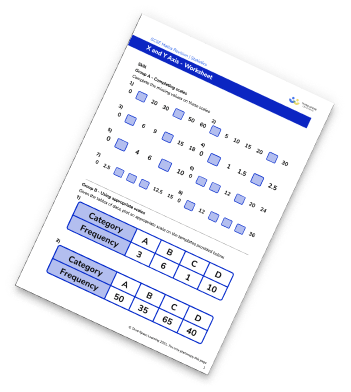Tally Charts Worksheet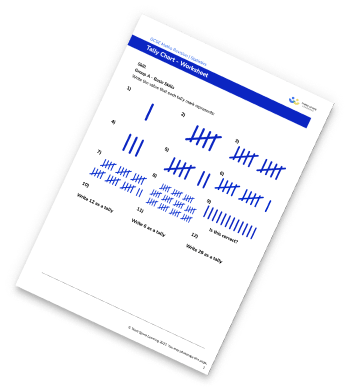Frequency graph worksheet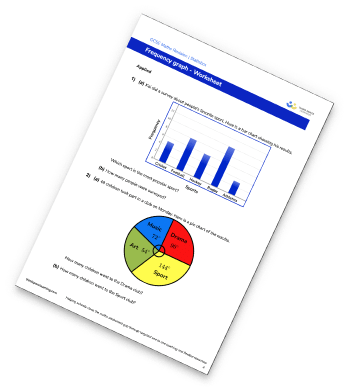Line Graphs Worksheet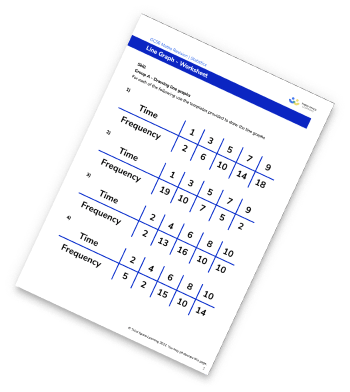## Do you have KS4 students who need more focused attention to succeed at GCSE?There will be students in your class who require individual attention to help them succeed in their maths GCSEs. In a class of 30, it’s not always easy to provide.

Help your students feel confident with exam-style questions and the strategies they’ll need to answer them correctly with our dedicated GCSE maths revision programme.

Lessons are selected to provide support where each student needs it most, and specially-trained GCSE maths tutors adapt the pitch and pace of each lesson. This ensures a personalised revision programme that raises grades and boosts confidence.

Find out more